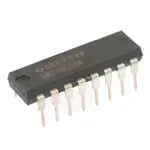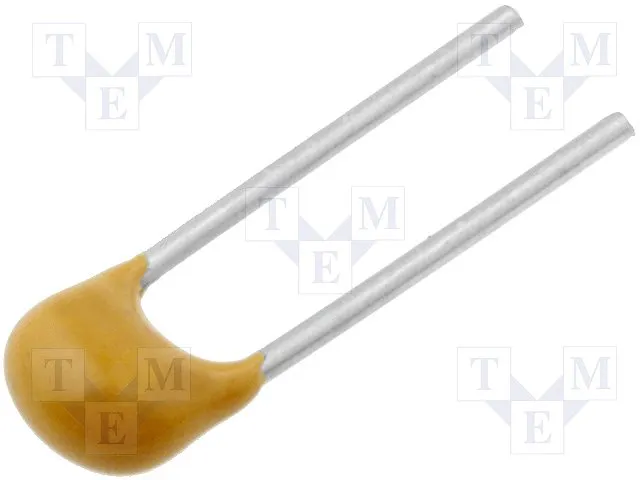# Using 74HC595 Shift Register

IntermediateFull instructions provided28

## Things used in this project

### Hardware componentsTexas Instruments Shift Register- Serial to Parallel
×1LED (generic)
×8Capacitor 100 nF
×1Arduino UNO & Genuino UNO
×1×1

## Code

### Code snippet #1

Plain text
```//Pin connected to ST_CP of 74HC595
int latchPin = 8;
//Pin connected to SH_CP of 74HC595
int clockPin = 12;
////Pin connected to DS of 74HC595
int dataPin = 11;

byte leds1 = 0b10101010;
byte leds2 = 0b01010101;

byte ledF1 = 0b10000001;
byte ledF2 = 0b01000010;
byte ledF3 = 0b00100100;
byte ledF4 = 0b00011000;

byte ledUp0 = 0b00000000;
byte ledUp1 = 0b10000000;
byte ledUp2 = 0b11000000;
byte ledUp3 = 0b11100000;
byte ledUp4 = 0b11110000;
byte ledUp5 = 0b11111000;
byte ledUp6 = 0b11111100;
byte ledUp7 = 0b11111110;
byte ledUp8 = 0b11111111;

void setup()
{
pinMode(latchPin, OUTPUT);
pinMode(dataPin, OUTPUT);
pinMode(clockPin, OUTPUT);
}

void loop()
{

// binaryNumberDisplay();
ledPattern();
//pingPong();
//backAndForth();

}

void backAndForth()
{
pushData(200, ledUp0);
pushData(200, ledUp1);
pushData(200, ledUp2);
pushData(200, ledUp3);
pushData(200, ledUp4);
pushData(200, ledUp5);
pushData(200, ledUp6);
pushData(200, ledUp7);
pushData(200, ledUp8);
pushData(200, ledUp7);
pushData(200, ledUp6);
pushData(200, ledUp5);
pushData(200, ledUp4);
pushData(200, ledUp3);
pushData(200, ledUp2);
pushData(200, ledUp1);
pushData(200, ledUp0);
}

void pingPong()
{
int delayTime = 200;
pushData(delayTime, ledF1);
pushData(delayTime, ledF2);
pushData(delayTime, ledF3);
pushData(delayTime, ledF4);
pushData(delayTime, ledF3);
pushData(delayTime, ledF2);
}

void ledPattern()
{
pushData(200, leds1);
pushData(200, leds2);
}

void binaryNumberDisplay()
{
for (int leds5 = 1; leds5 < 255; leds5++)
{
pushData(500, leds5);
}
}

void pushData(int delayTime, byte data)
{
delay(delayTime);
digitalWrite(latchPin, LOW);
shiftOut(dataPin, clockPin, LSBFIRST, data);
digitalWrite(latchPin, HIGH);
}
```

### Code snippet #2

Plain text
```//Pin connected to ST_CP of 74HC595
int latchPin = 8;
//Pin connected to SH_CP of 74HC595
int clockPin = 12;
////Pin connected to DS of 74HC595
int dataPin = 11;

byte leds1 = 0b10101010;
byte leds2 = 0b01010101;

byte ledF1 = 0b10000001;
byte ledF2 = 0b01000010;
byte ledF3 = 0b00100100;
byte ledF4 = 0b00011000;

byte ledUp0 = 0b00000000;
byte ledUp1 = 0b10000000;
byte ledUp2 = 0b11000000;
byte ledUp3 = 0b11100000;
byte ledUp4 = 0b11110000;
byte ledUp5 = 0b11111000;
byte ledUp6 = 0b11111100;
byte ledUp7 = 0b11111110;
byte ledUp8 = 0b11111111;

void setup()
{
pinMode(latchPin, OUTPUT);
pinMode(dataPin, OUTPUT);
pinMode(clockPin, OUTPUT);
}

void loop()
{

// binaryNumberDisplay();
ledPattern();
//pingPong();
//backAndForth();

}

void backAndForth()
{
pushData(200, ledUp0);
pushData(200, ledUp1);
pushData(200, ledUp2);
pushData(200, ledUp3);
pushData(200, ledUp4);
pushData(200, ledUp5);
pushData(200, ledUp6);
pushData(200, ledUp7);
pushData(200, ledUp8);
pushData(200, ledUp7);
pushData(200, ledUp6);
pushData(200, ledUp5);
pushData(200, ledUp4);
pushData(200, ledUp3);
pushData(200, ledUp2);
pushData(200, ledUp1);
pushData(200, ledUp0);
}

void pingPong()
{
int delayTime = 200;
pushData(delayTime, ledF1);
pushData(delayTime, ledF2);
pushData(delayTime, ledF3);
pushData(delayTime, ledF4);
pushData(delayTime, ledF3);
pushData(delayTime, ledF2);
}

void ledPattern()
{
pushData(200, leds1);
pushData(200, leds2);
}

void binaryNumberDisplay()
{
for (int leds5 = 1; leds5 < 255; leds5++)
{
pushData(500, leds5);
}
}

void pushData(int delayTime, byte data)
{
delay(delayTime);
digitalWrite(latchPin, LOW);
shiftOut(dataPin, clockPin, LSBFIRST, data);
digitalWrite(latchPin, HIGH);
}
```

### Code to blink LEDs in different pattern using shift register

C/C++
Code to blink LEDs in different pattern using shift register
```//Pin connected to ST_CP of 74HC595
int latchPin = 8;
//Pin connected to SH_CP of 74HC595
int clockPin = 12;
////Pin connected to DS of 74HC595
int dataPin = 11;

byte leds1 = 0b10101010;
byte leds2 = 0b01010101;

byte ledF1 = 0b10000001;
byte ledF2 = 0b01000010;
byte ledF3 = 0b00100100;
byte ledF4 = 0b00011000;

byte ledUp0 = 0b00000000;
byte ledUp1 = 0b10000000;
byte ledUp2 = 0b11000000;
byte ledUp3 = 0b11100000;
byte ledUp4 = 0b11110000;
byte ledUp5 = 0b11111000;
byte ledUp6 = 0b11111100;
byte ledUp7 = 0b11111110;
byte ledUp8 = 0b11111111;

void setup()
{
pinMode(latchPin, OUTPUT);
pinMode(dataPin, OUTPUT);
pinMode(clockPin, OUTPUT);
}

void loop()
{

// binaryNumberDisplay();
ledPattern();
//pingPong();
//backAndForth();

}

void backAndForth()
{
pushData(200, ledUp0);
pushData(200, ledUp1);
pushData(200, ledUp2);
pushData(200, ledUp3);
pushData(200, ledUp4);
pushData(200, ledUp5);
pushData(200, ledUp6);
pushData(200, ledUp7);
pushData(200, ledUp8);
pushData(200, ledUp7);
pushData(200, ledUp6);
pushData(200, ledUp5);
pushData(200, ledUp4);
pushData(200, ledUp3);
pushData(200, ledUp2);
pushData(200, ledUp1);
pushData(200, ledUp0);
}

void pingPong()
{
int delayTime = 200;
pushData(delayTime, ledF1);
pushData(delayTime, ledF2);
pushData(delayTime, ledF3);
pushData(delayTime, ledF4);
pushData(delayTime, ledF3);
pushData(delayTime, ledF2);
}

void ledPattern()
{
pushData(200, leds1);
pushData(200, leds2);
}

void binaryNumberDisplay()
{
for (int leds5 = 1; leds5 < 255; leds5++)
{
pushData(500, leds5);
}
}

void pushData(int delayTime, byte data)
{
delay(delayTime);
digitalWrite(latchPin, LOW);
shiftOut(dataPin, clockPin, LSBFIRST, data);
digitalWrite(latchPin, HIGH);
}
```

## Credits

### aaravpatel0124

2 projects • 0 followers